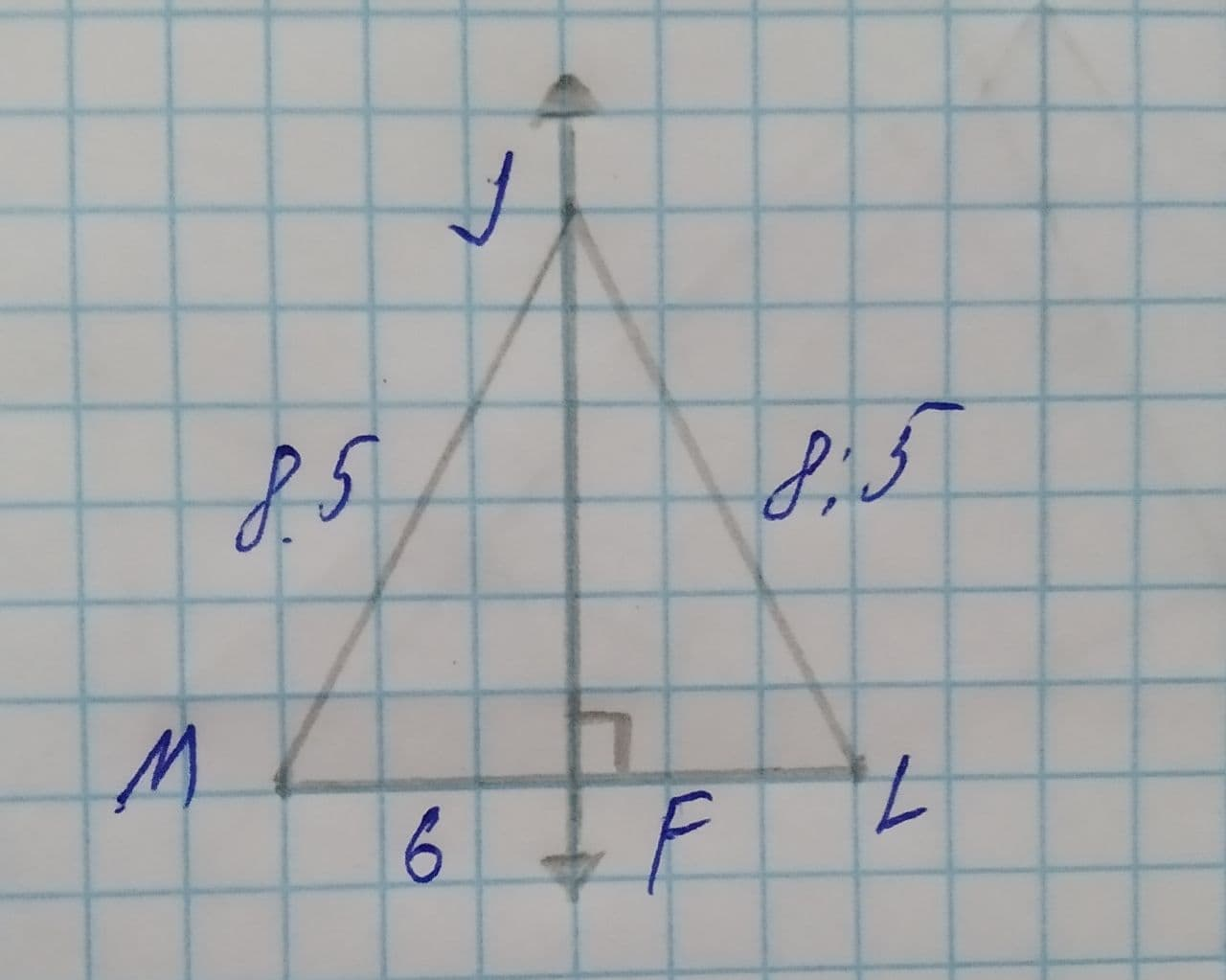hexacordoK

2021-05-09

To find: The value of measure LM.comentezq

Given:
The given figure is as follows.Calculation:
As shown in the figure $\stackrel{―}{JM}\stackrel{\sim }{=}\stackrel{―}{JL}$.
From the Reflexive Property of congruence, $\stackrel{―}{JF}\stackrel{\sim }{=}\stackrel{―}{JF}$.
The $\mathrm{\angle }JFM$, and $\mathrm{\angle }JFL$ right anges because $\stackrel{―}{JF}perp\stackrel{―}{ML}$.
From the HL congruence, $\mathrm{△}JFM\stackrel{\sim }{=}\mathrm{△}JFL$.
In congruent triangles, corresponding elemnts are congruent so $\stackrel{―}{MF}\stackrel{\sim }{=}\stackrel{―}{LF}$.
From the definition of congruent segments MF =LF =6.
LM=MF+LF
=6+6
=12
Therefore, the value of measure LM is 12.

Do you have a similar question?